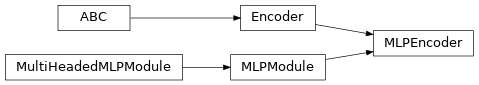# `garage.torch.embeddings`¶

PyTorch embedding modules for meta-learning algorithms.

class MLPEncoder(input_dim, output_dim, hidden_sizes, hidden_nonlinearity=F.relu, hidden_w_init=nn.init.xavier_normal_, hidden_b_init=nn.init.zeros_, output_nonlinearity=None, output_w_init=nn.init.xavier_normal_, output_b_init=nn.init.zeros_, layer_normalization=False)This MLP network encodes context of RL tasks.

Context is stored in the terms of observation, action, and reward, and this network uses an MLP module for encoding it.

Parameters
• input_dim (int) – Dimension of the network input.

• output_dim (int) – Dimension of the network output.

• hidden_sizes (list[int]) – Output dimension of dense layer(s). For example, (32, 32) means this MLP consists of two hidden layers, each with 32 hidden units.

• hidden_nonlinearity (callable or torch.nn.Module) – Activation function for intermediate dense layer(s). It should return a torch.Tensor.Set it to None to maintain a linear activation.

• hidden_w_init (callable) – Initializer function for the weight of intermediate dense layer(s). The function should return a torch.Tensor.

• hidden_b_init (callable) – Initializer function for the bias of intermediate dense layer(s). The function should return a torch.Tensor.

• output_nonlinearity (callable or torch.nn.Module) – Activation function for output dense layer. It should return a torch.Tensor. Set it to None to maintain a linear activation.

• output_w_init (callable) – Initializer function for the weight of output dense layer(s). The function should return a torch.Tensor.

• output_b_init (callable) – Initializer function for the bias of output dense layer(s). The function should return a torch.Tensor.

• layer_normalization (bool) – Bool for using layer normalization or not.

property spec

Input and output space.

Type

garage.InOutSpec

property input_dim

Dimension of the encoder input.

Type

int

property output_dim

Dimension of the encoder output (embedding).

Type

int

reset(do_resets=None)

Reset the encoder.

This is effective only to recurrent encoder. do_resets is effective only to vectoried encoder.

For a vectorized encoder, do_resets is an array of boolean indicating which internal states to be reset. The length of do_resets should be equal to the length of inputs.

Parameters

do_resets (numpy.ndarray) – Bool array indicating which states to be reset.

forward(input_value)

Forward method.

Parameters

input_value (torch.Tensor) – Input values with (N, *, input_dim) shape.

Returns

Output value

Return type

torch.Tensor